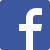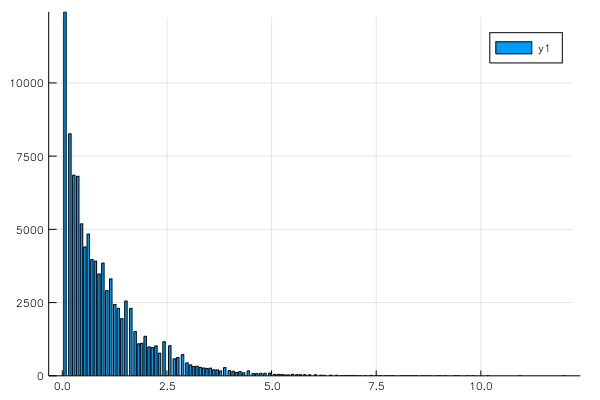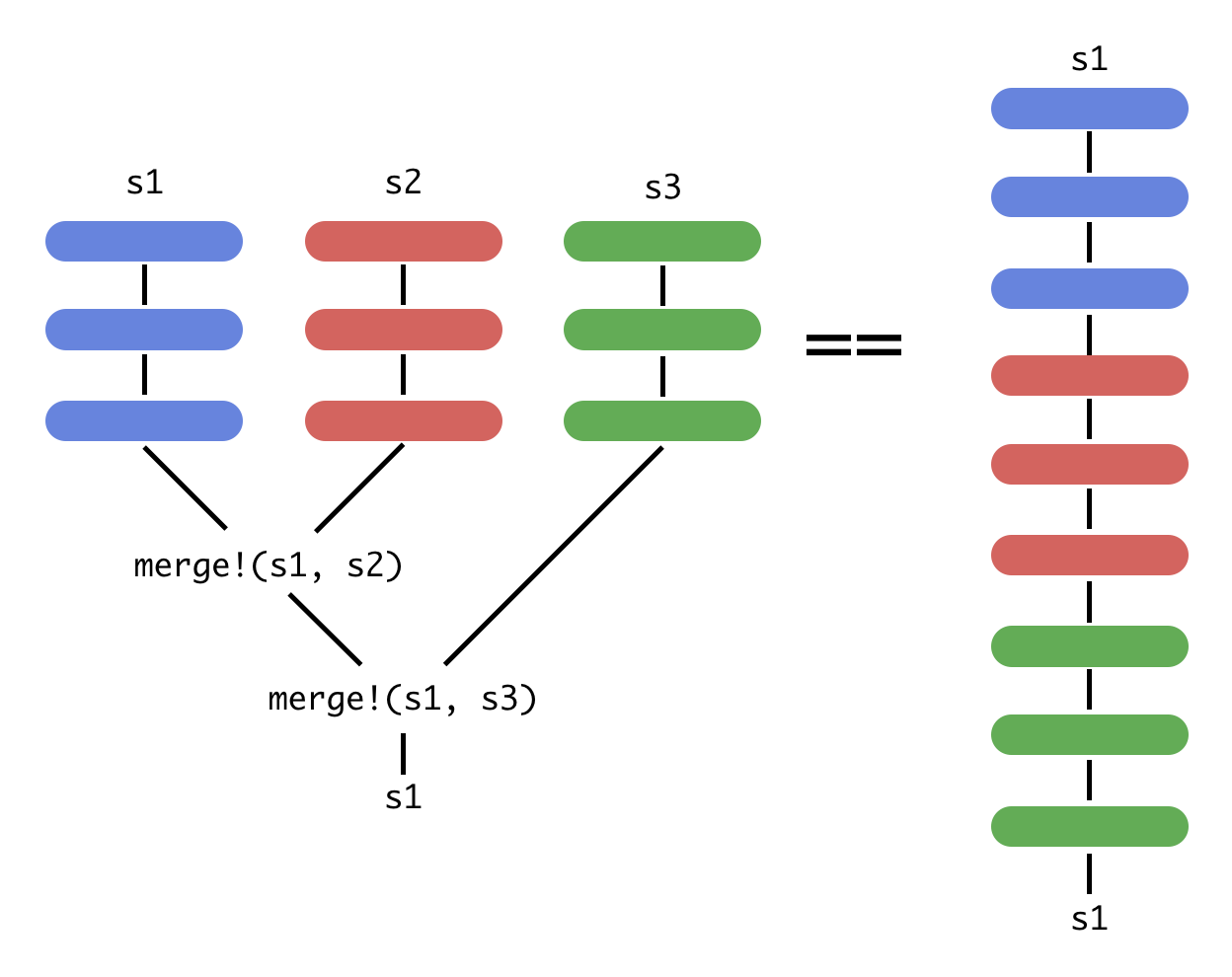Here is the latest from Julia Computing
BLG# Big Data Analytics with OnlineStats.jl

16 Nov 2017 | Josh Day

OnlineStats is a package for computing statistics and models via online algorithms. It is designed for taking on big data and can naturally handle out-of-core processing, parallel/distributed computing, and streaming data. JuliaDB fully integrates OnlineStats for providing analytics on large persistent datasets. While future posts will dive into this integration, this post serves as a light introduction to OnlineStats.

## What are Online Algorithms?

Online algorithms accept input one observation at a time. Consider a mean of n data points:

By adding a single observation, the mean could be recalculated from scratch (offline):

Or we could use only the current estimate and the new observation (online):

A big advantage of online algorithms is that data does not need to be revisited when new observations are added. It is therefore not necessary for the dataset to be fixed in size or small enough to fit in computer memory. The disadvantage is that not everything can be calculated exactly like the mean above. Whenever exact solutions are impossible, OnlineStats relies on state of the art stochastic approximation algorithms.

## OnlineStats Basics

The statistics/models of OnlineStats are subtypes of OnlineStat:

using OnlineStats, Plots

# Each OnlineStat is a type
o = IHistogram(100)
o2 = Sum()

# OnlineStats are grouped together in a Series
s = Series(o, o2)

# Updating the Series updates the grouped OnlineStats
y = randexp(100_000)

# fit!(s, y) translates to:
for yi in y
fit!(s, yi)
end

plot(o)## Working with Series of Different Inputs

A Series groups together any number of OnlineStats which share a common input. The input (single observation) of an OnlineStat can be a scalar (e.g. Variance), a vector (e.g. CovMatrix), or a vector/scalar pair (e.g. LinReg).

The Series constructor optionally accepts data to fit! right away.

• Scalar-input Series
julia> Series(randn(100), Mean(), Variance())
▦ Series{0} with EqualWeight
├── nobs = 100
├── Mean(0.0899071)
└── Variance(0.952008)

• Vector-input Series
• The MV type can turn a scalar-input OnlineStat into a vector-input version.
julia> Series(randn(100, 2), CovMatrix(2), MV(2, Mean()))
▦ Series{1} with EqualWeight
├── nobs = 100
├── CovMatrix([0.916472 0.089655; 0.089655 0.984442])
└── MV{Mean}(0.17287277199330608, -0.12199728546589127)

• Vector/Scalar-input Series
• The Vector holds predictor variables and the Scalar is a response.
julia> Series((randn(100, 3), randn(100)), LinReg(3))
▦ Series{(1, 0)} with EqualWeight
├── nobs = 100
└── LinReg: β(0.0) = [-0.0486756 -0.0437766 -0.160813]


## Working with Series and Individual OnlineStats

• value returns the stat’s value
julia> o = Mean()
Mean(0.0)

julia> value(o)
0.0

• value on a Series maps value to the stats
julia> s = Series(Mean(), Variance())
▦ Series{0} with EqualWeight
├── nobs = 0
├── Mean(0.0)
└── Variance(-0.0)

julia> value(s)
(0.0, -0.0)

• stats returns a tuple of stats
julia> m, v = stats(s)
(Mean(0.0), Variance(-0.0))


## (Embarassingly) Parallel Computation

At first glance, it appears necessary that a Series must be fit!t-ed serially, but OnlineStats provides merge/merge! methods for combining two Series into one. This is how JuliaDB is able to use OnlineStats in a distributed fashion. Below is a simple (not actually parallel) example of merging.

s1 = Series(Mean(), Variance())
s2 = Series(Mean(), Variance())
s3 = Series(Mean(), Variance())

fit!(s1, randn(1000))
fit!(s2, randn(1000))
fit!(s3, randn(1000))

merge!(s1, s2)
merge!(s1, s3)# Resources

This is a small sample of OnlineStats functionality. For more information, stay tuned for future posts or check out the OnlineStats Github repo and documentation.

## Recent posts

CSV Reader Benchmarks: Julia Reads CSVs 10-20x Faster than Python and R
22 Jun 2020 | Deepak Suresh
JuliaTeam v2.0 Now Available
12 Jun 2020 | Julia Computing